# 一、推荐系统架构与机器学习基础理论

## 1 机器学习发展历程与架构原理

### 1.1 发展历程

• 大数据时代： 频率近似为概率

• 计算机性能 ：计算速度提升

### 1.2 优化问题与凸优化问题

• 任何的机器学习问题都可以归结于优化问题

• 任何优化问题都可以具体为多个凸优化

• 优化问题：也叫最优化问题，是指在一定约束条件下，求解一个目标函数的最大值（或最小值）问题。

• 优化问题一般都是通过迭代的方式来求解：通过猜测一个初始的估计值，然后不断迭代产生新的估计值，最终收敛到期望的最优解。一个好的优化算法应该是在 一定的时间或空间复杂度下能够快速准确地找到最优解。
• 凸优化：凸优化是指一种比较特殊的优化，是指求取最小值的目标函数为凸函数的一类优化问题。

• 目的是求取目标函数的最小（优）值；
• 目标函数和不等式约束函数都是凸函数，定义域是凸集；
• 若存在等式约束函数，则等式约束函数为仿射函数；
• 对于凸优化问题具有良好的性质，局部最优解便是全局最优解。

### 1.3 推荐系统的背景

• 分类导航：信息缺乏，用户主动寻找信息

• 搜索引擎：信息丰富，用户主动寻找信息

• 推荐系统：信息泛滥，信息寻找用户

#### 1.3.1 搜索引擎与推荐系统的区别

• 搜索引擎：满足用户有明确目的时主动查找的需求

• 推荐系统：帮助用户在没有明确目的时，发现感兴趣的内容

### 1.4 推荐系统的构成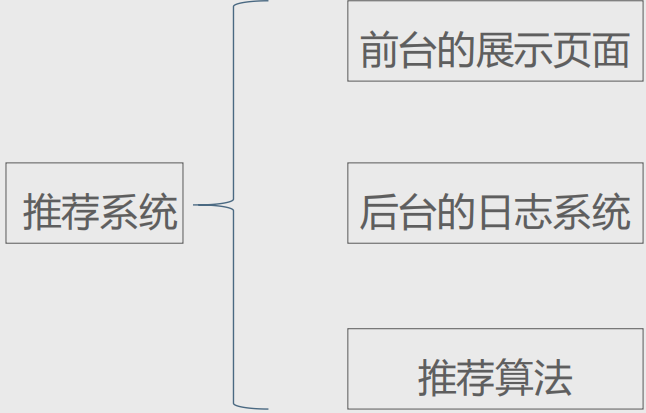• 前台为我们展示信息，会让你对这个信息进行打分，点赞，关注等
• 后台的日志系统会把你的这些操作录入日志中
• 最后我们的推荐算法，通过对你的打分，关注等的信息进行计算，为你推荐一些相同的信息

### 1.5 推荐系统的架构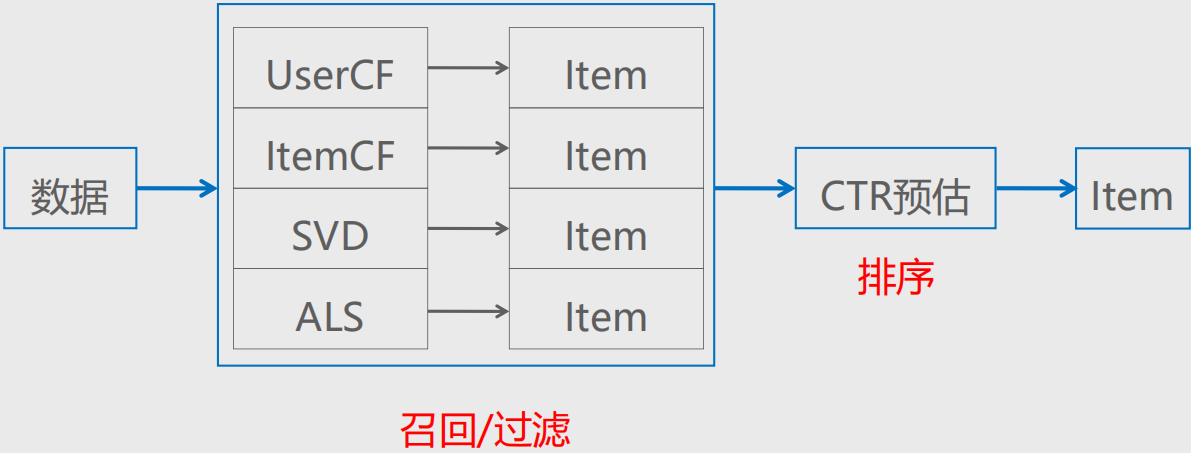• 推荐系统通用架构图，主要包括：底层基础数据、数据加工存储、召回内容、计算排序、过滤和展示、业务应用。
• 底层基础数据是推荐系统的基石，只有数据量足够多，才能从中挖掘出更多有价值的信息，进而更好地为推荐系统服务。
• 我们的数据量很大，并不能直接把所有的物品数据全部输送到推荐系统进行排序。在进行物品召回时可以基于一些常用的机器学习算法构建用户偏好模型、用户兴趣模型、物品相似模型、物品互补模型等。在进行内容召回时，只召回和用户有偏好关系、和用户有直接关联、和用户有直接关系的相关物品，输入排序模型，进行打分排序
• 在得到召回的物品之后，就要考虑如何对这些物品进行正确的排序。目前业界在机器学习领域最普遍的做法是将排序推荐模型作为二分类模型来训练，即在构造样本集的过程中对应的标签为0或1（未点击或点击）。常用的排序算法包括但不局限于GBDT、LR、XGBoost等
• 过滤和展示直接影响用户体验，因此在做推荐系统时一定要注意相关的过滤和展示规则。

## 2 80/20原则与长尾效应

### 2.1 80/20原则

80和20是两种不同事物的比重，因此没有合计为100的说法。前者是销量的比重，后者是产品的比重。 更糟糕的是，对于如何表达两者之间的关系，或是将哪一个变量定为常量， 我们并没有标准的惯例。你可以说一个市场呈 现出80/10的形状( 10%的产品带来80%的销量)，也可以说它的形状是95/20 (20%的产品带来95%的销量)。人们用这个法则来解释截然不同的现象。80和20的经典定义是产品和收益，但这个法则同样可以应用于产品和利润的关系。

### 2.2 长尾效应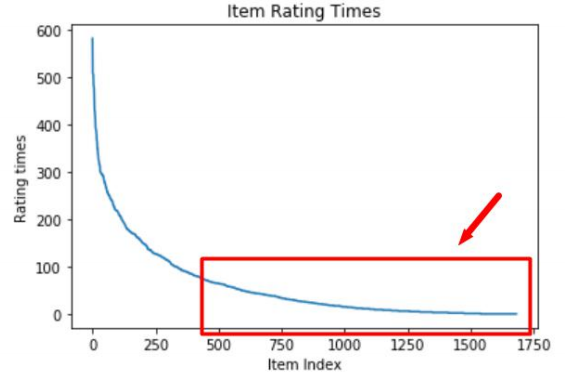#### 2.2.1 解决思路

• 用户分析

• 新用户：倾向于浏览热门商品
• 老用户：逐渐开始浏览冷门物品
• 新颖度

• 覆盖度

## 3 新算法上线流程与用户满意度收集策略

### 3.1 新算法上线流程

• ##### 离线实验
• 日志生成标准数据集
• 数据集分为训练集和测试集
• 训练集上训练模型，测试集上进行预测
• 根据指标，评价预测结果
• ##### 用户调查
• 高预测准确率不等于用户满意度
• 算法上线测试之前需要真实用户来参与测试
• 注： 要保证参与测试的用户与实际用户分布相同
• ##### 在线AB测试
• AB测试：新算法与旧算法进行比较
• 注： 在线实验的成本更高，只有离线实验和用户调查中表现都很 好的算法才可以进行
• 新算法最终上线的条件：

• 在离线指标上优于现有算法
• 用户调查满意度不低于现有算法
• 在线AB测试结果优于现有算法

• 调查问卷

• 点击率

• 用户停留时间

• 转化率

• 标签反馈

## 4 RMSE与MAE评价准确度

### 4.1 RMSE和MAE

MAE和RMSE是关于连续变量的两个最普遍的度量标准。

MSE:均方误差是指参数估计值与参数真值之差平方的期望值; MSE可以评价数据的变化程度，MSE的值越小，说明预测模型描述实验数据具有更好的精确度。 MAE是一种线性分数，所有个体差异在平均值上的权重都相等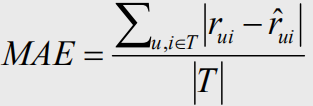RMSE:均方误差，均方根误差是均方误差的算术平方根，表示预测值和观测值之间差异（称为残差）的样本标准偏差。 均方根误差为了说明样本的离散程度。做非线性拟合时，RMSE越小越好。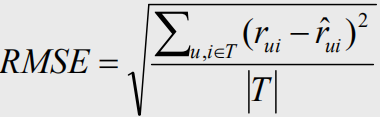• RMSE加大了预测不准评分的惩罚

### 4.2 准确率与召回率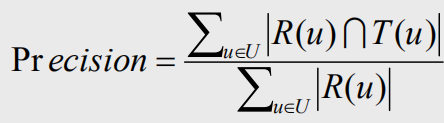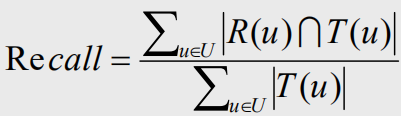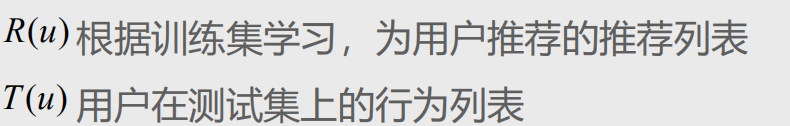• 准确率：为用户推荐的，有多少是用户感兴趣的

• 召回率：用户感兴趣的，有多少被推荐了

## 5 信息熵与基尼系数衡量覆盖率

### 5.1 信息量

#### 5.1.1 信息熵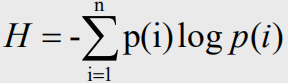#### 5.1.2 信息熵的性质

• 单调性，发生概率越高的事件，其携带的信息量越低；

• 非负性，信息熵可以看作为一种广度量，非负性是一种合理的必然；

• 累加性，即多随机事件同时发生存在的总不确定性的量度是可以表示为各事件不确定性的量度的和，这也是广度量的一种体现。

### 5.2 基尼系数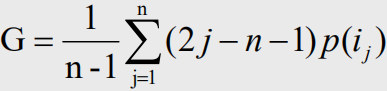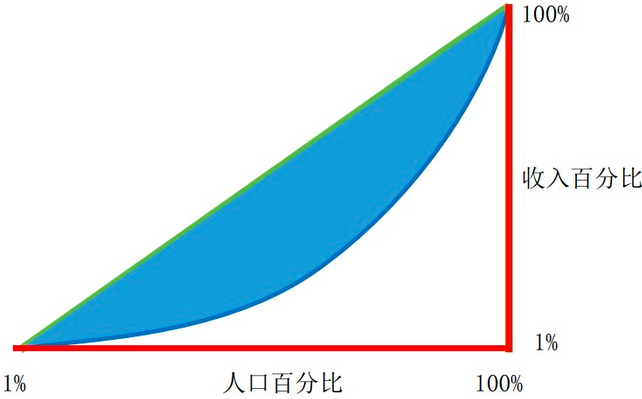### 5.3 覆盖度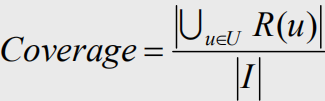import pandas as pd
import numpy as np
import matplotlib.pyplot as plt

# 读取rating.csv，读取的列为：userId，movieId，rating
# 打印前5行

# 每个电影id对应的打分次数
movie_rating_count = df_data['movieId'].value_counts()
# 打印前5行

# 深度拷贝一个movie_rating_count
movie_rating_count2  = movie_rating_count.copy()
# 重置索引，方便画图
movie_rating_count2.index = range(movie_rating_count.count())
# 横坐标为充值后的索引，纵坐标为打分次数
plt.plot(movie_rating_count2.index,movie_rating_count2)
plt.xlabel('Rating Count')
plt.ylabel('Movie Index')
plt.title('Movie Rating Count')
plt.show()

print(movie_rating_count.tail())
# 数据集中出现的最大的电影id
movie_id_max = movie_rating_count.index.max()
print('最大的商品id：',movie_id_max)

# 总打分数
total_rating_count = sum(movie_rating_count)
print('总打分数是：',total_rating_count)

# 参与评论的电影数量：movie_quantity = len(movie_rating_count)也可以
movie_quantity = movie_rating_count.count()
print('参与评论的电影数量：',movie_quantity)


# 计算信息熵
h = 0
for rating_count in movie_rating_count:
p = rating_count / total_rating_count
logp = np.log(p)
h += -1 * p * logp
print(h)


# 计算基尼指数
gini_index = 0
for index in range(len(movie_rating_count)):
p = movie_rating_count.iloc[index] / total_rating_count
# j是根据流行度从小到大排列的列表中，第j个电影，而movie_rating_count中是根据打分次数从大到小排列的
j = movie_quantity - index
gini_index += (2 * j - movie_quantity -1) * p
gini_index = gini_index / (movie_quantity - 1)
print(gini_index)

# 计算覆盖度，这里把电影id的最大值记为总电影数目，把评分过的电影，记为推荐过的电影
coverage = movie_quantity / movie_id_max
print(coverage)


## 6 多样性的度量 —— 相似度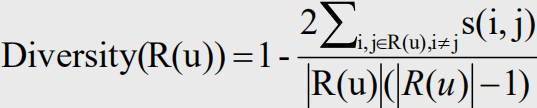s(i,j) 物品i与物品j的相似度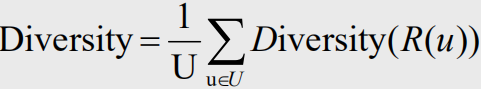• 个体多样性 从单个用户的角度来度量推荐的多样性主要考察系统能够找到用户喜欢的冷门项目的能力。个性化推荐多样化的目标就

• 与个体多样性不同,总体多样性主要强调针对不同用户的推荐应尽可能地不同

• 将时间情境融入到推荐系统中,构建用户——项目——时间三维推荐模型,可以有效提高个性化推荐的时序多样性。

## 7 抵御行为注入攻击策略

### 7.1 行为注入攻击

• 想让自己的店铺在商城网站中排名靠前：

• 雇佣一些账号来购买本店铺商品，私下里退款
• 雇佣购买过一些热门商品的用户，再购买本店铺的商品
• 通过增加评分提高推荐的权重：

• 例如豆瓣电影，雇佣水军对电影打高分

### 7.2 行为注入攻击的防范

相似度度量是协同过滤算法的核心模块，但易于遭受推荐攻击问题。近年来，信誉模型被融合到推荐流程中，加强协同过滤算法的鲁棒性和推荐精确性。

• 防范方式：
• 使用高代价的用户行为
• 使用数据前进行攻击检测，对数据清理

# 二、协同过滤算法实战与优化

## 1 基于用户的协同过滤算法

### 1.1 UserCF 算法

UserCF 基于用户的协同过滤算法

CF算法的原理，汇总所有<user,item>的行为对, 利用集体智慧做推荐。其原理很像朋友推荐, 比如通过对用户喜欢的item进行分析, 发现用户A和用户B很像(他们都喜欢差不多的东西), 用户B喜欢了某个item, 而用户A没有喜欢, 那么就把这个item推荐给用户A。

• 找到和目标用户兴趣相似的用户集合

• 计算两个用户的兴趣相似度。这里，协同过滤算法主要利用行为的相似度计算兴趣的相似度。给定用户u和用户v，用户u曾经有过正反馈的物品集合，用户v曾经有过正反馈的物品集合。那么，我们可以通过如下公式简单地计算u和v的兴趣相似度
• 找到这个集合中的用户喜欢的，且目标用户没有听说过的物品推荐给目标用户。

• 得到用户之间的兴趣相似度后，UserCF算法会给用户推荐和他兴趣最相似的K个用户喜欢的物品。
• 算法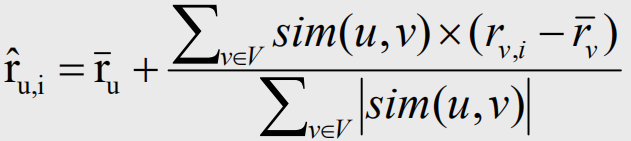import numpy as np
# 用户计算距离
import scipy.spatial.distance as ssd

#|用户|商品1|商品2|商品3|商品4|商品5|
#|:---|:---|:---|:---|:---|:---|
#|用户1|1分|4分|2分|1分|？|
#|用户2|2分|4分|2分|1分|5分|
#|用户3|5分|1分|5分|4分|2分|
#|用户4|2分|5分|3分|4分|5分|

# 用户特征向量
r1 = np.array([1, 4, 2, 1])
r2 = np.array([2, 4, 2, 1])
r3 = np.array([5, 1, 5, 4])
r4 = np.array([2, 5, 3, 4])

# Pearson相关系数
def sim(v1,v2):
# 用户1和用户2的平均打分
v1_mu = sum(v1) / len(v1)
v2_mu = sum(v2) / len(v2)
# 特征向量去均值
v1_ = v1 - v1_mu
v2_ = v2 - v2_mu
# 返回相关系数
return 1 - ssd.cosine(v1_,v2_)

# 计算用户1和2、3、4用户的相关系数
sim1_2 = sim(r1, r2)
sim1_3 = sim(r1, r3)
sim1_4 = sim(r1, r4)
print('用户1与用户2的相似度：', sim1_2)
print('用户1与用户3的相似度：', sim1_3)
print('用户1与用户4的相似度：', sim1_4)

# 计算用户打分均值
r1_mu = sum(r1) / len(r1)
r2_mu = sum(r2) / len(r2)
r3_mu = sum(r3) / len(r3)
r4_mu = sum(r4) / len(r4)

# 带入公式，预测打分
predict_rating = sum(r1)/len(r1) + ((5) * sim1_2 + 2 * sim1_3 + 5 * sim1_4) / (np.abs(sim1_2) + np.abs(sim1_3) + np.abs(sim1_4))
print('计算后的预测分数是：',predict_rating)

# 越界问题，评分最低0分，最高5分
# predict_rating > 5  --> predict_rating=5
# predict_rating < 0  --> predict_rating=0
predict_rating = np.clip(predict_rating, 0, 5)
print('最终预测打分：', predict_rating)


### 1.2 ItemCF 算法

• 计算物品之间的相似度

• 就是假设每个用户的兴趣都局限在某几个方面，因此如果两个物品属于一个用户的兴趣列表，那么这两个物品可能就属于有限的几个领域，而如果两个物品属于很多用户的兴趣列表，那么它们就可能属于同一个领域，因而有很大的相似度。
• 根据物品的相似度和用户的历史行为给用户生成推荐列表

• 用户历史上感兴趣的物品越相似的物品，越有可能在用户的推荐列表中获得比较高的排名。
• 算法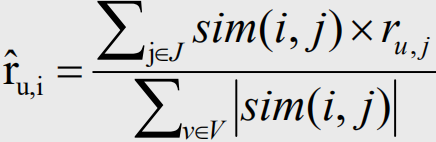import numpy as np
import scipy.spatial.distance as ssd
import pandas as pd

# 读取数据
df_data.index = ['user1', 'user2', 'user3', 'user4']

# user2到user4的平均值向量
user_mu = [
sum(df_data.loc[index])/len(df_data.loc[index])
for index in df_data.iloc[1:4].index
]
user_mu

# 相关系数函数
def sim(v1,v2):
# 去均值
v1_ = v1 - user_mu
v2_ = v2 - user_mu
return 1 - ssd.cosine(v1_, v2_)
# 得到item1到item5的相关系数向量
sim_v = np.array(
[ sim(df_data['item5'][1:4], df_data[item][1:4])
for item in ['item1', 'item2', 'item3', 'item4']
]
)
sim_v

# 预测打分
predict_rating = np.dot(sim_v,df_data.iloc[0:4]) / sum(np.abs(sim_v))
print('计算后的预测分数是：', predict_rating)

predict_rating = np.clip(predict_rating,0,5)
print('最终预测打分：', predict_rating)


### 1.3 UserCF 与 ItemCF的区别

• user和item数量分布以及变化频率

• 如果user数量远远大于item数量, 采用Item-CF效果会更好, 因为同一个item对应的打分会比较多, 而且计算量会相对较少
• 如果item数量远远大于user数量, 则采用User-CF效果会更好, 原因同上
• 如果用户行为变化频率很慢(比如小说), 用User-CF结果会比较稳定
• 如果用户行为变化频率很快(比如新闻, 音乐, 电影等), 用Item-CF结果会比较稳定
• 相关和惊喜的权衡

• item-based出的更偏相关结果, 出的可能都是看起来比较类似的结果
• user-based出的更有可能有惊喜, 因为看的是人与人的相似性, 推出来的结果可能更有惊喜
• 数据更新频率和时效性要求

• 对于item更新时效性较高的产品, 比如新闻, 就无法直接采用item-based的CF, 因为CF是需要批量计算的, 在计算结果出来之前新的item是无法被推荐出来的, 导致数据时效性偏低;
• 但是可以采用user-cf, 再记录一个在线的用户item行为对, 就可以根据用户最近类似的用户的行为进行时效性item推荐;
• 对于像影视, 音乐之类的还是可以采用item-cf的

## 2 Movielens电影推荐数据分析

MovieLens数据集包含多个用户对多部电影的评级数据，也包括电影元数据信息和用户属性信息。这个数据集经常用来做推荐系统，机器学习算法的测试数据集。尤其在推荐系统领域，很多著名论文都是基于这个数据集的。

### 2.1 UserCf 推荐Movielen

#### 2.1.1 读取训练集

import pandas as pd
import numpy as np
import scipy.spatial.distance as ssd
from collections import defaultdict

# 读取训练集，使用到的列为user_id，item_id，rating
'./data/movielen_rating_training.base',
names=['user_id','item_id','rating'],
usecols=[0,1,2],
sep='\t')

# 不重复的user_id与item_id列表
user_id_s = df_training_data['user_id'].unique()
item_id_s = df_training_data['item_id'].unique()
# 建立id与index的索引
# 例如：user_id_map[【user_id】] = user_index
user_index_map = {}
item_index_map = {}
for user_index in range(len(user_id_s)):
user_id = user_id_s[user_index]
user_index_map[user_id] = user_index
for item_index in range(len(item_id_s)):
item_id = item_id_s[item_index]
item_index_map[item_id] = item_index

# 用户与物品的打分矩阵
user_item_rating_array = np.zeros(shape=(len(user_id_s),len(item_id_s)))
# 用户打分商品的索引集合
user_rating_map = defaultdict(set)

for row_index in df_training_data.index:
# 每一行的数据
row_data = df_training_data.iloc[row_index]
# 打分用户的索引
user_index = user_index_map[row_data['user_id']]
# 打分电影的索引
item_index = item_index_map[row_data['item_id']]
# 添加用户打分商品索引集合
# 矩阵中行=user_index，列=item_index的元素赋值为打分
user_item_rating_array[user_index,item_index] = row_data['rating']
user_item_rating_array

# 计算用户的平均打分向量
def calculate_user_rating_mu():
user_rating_mu_s = []
for user_index in range(len(user_id_s)):
# 计算打过分的电影索引
item_rating_index_v = list(user_rating_map[user_index])
# 打过分的电影评分向量
item_rating_v = np.take(
user_item_rating_array[user_index],
item_rating_index_v
)
# 打分向量的平均值
mu = item_rating_v.mean()
# 保留两位小数
mu = round(mu,2)
user_rating_mu_s.append(mu)
return user_rating_mu_s
# ----------------【也可以写成一句话】----------------------------
# def calculate_user_rating_mu():
#     return [
#         round(
#             np.take(
#                 user_item_rating_array[user_index],
#                 list(user_rating_map[user_index])
#             ).mean(),
#             2
#         )
#         for user_index in range(len(user_id_s))
#     ]
# -----------------------------------------------------------------
# 得到用户打分的平均值，列表类型，列表索引对应user_index
user_rating_mu_s = calculate_user_rating_mu()
user_rating_mu_s


#### 2.1.2 相似度函数

# 定义用户相似度函数
def calculate_sim(user_index1,user_index2):
# 取用户1和用户2公共打分的电影集合，转换成列表
intersection_index_s = list(
user_rating_map[user_index1] & user_rating_map[user_index2]
)
# 如果没有公共的打分项，相似度为0.0
if not intersection_index_s:
return 0.0
# 根据公共索引，取到用户1的打分向量，并去均值
v1 = np.take(
user_item_rating_array[user_index1],
intersection_index_s
) - user_rating_mu_s[user_index1]
# 根据公共索引，取到用户2的打分向量，并去均值
v2 = np.take(
user_item_rating_array[user_index2],
intersection_index_s
) - user_rating_mu_s[user_index2]
# 计算相似度
sim = 1 - ssd.cosine(v1,v2)
# 如果相似度不是数字（如果v1或v2是0向量），返回相似度0
if np.isnan(sim):
return 0.0
# 否则相似度保留两位小数，返回结果
else:
return round(sim,2)

user_similarity_array = np.zeros(shape=(len(user_id_s),len(user_id_s)))
for user_index1 in range(len(user_id_s)):
print('计算到用户：%s与其余用户的相似度' % user_index1)
for user_index2 in range(user_index1 + 1,len(user_id_s)):
# 计算用户1和用户2的相似度
sim = calculate_sim(user_index1,user_index2)
# 用户1与用户2的相似度=sim
user_similarity_array[user_index1,user_index2] = sim
# 用户2与用户1的相似度=sim
user_similarity_array[user_index2,user_index1] = sim

# 打印用户相似度矩阵
user_similarity_array


#### 2.1.3 预测用户

# 用户对商品的预测矩阵，已打分的商品，预测分数为0分
user_item_predict_rating_array = np.zeros_like(user_item_rating_array)

# 对所有的用户index进行遍历
for user_index in range(len(user_id_s)):
print('预测到用户%s' % user_index)
# 对所有商品进行遍历
for item_index in range(len(item_id_s)):
# 如果这个商品没有被打过分
if item_index not in user_rating_map[user_index]:
# 找到对这个商品打过分的所有用户的索引
user_rating_index_v = np.where(
user_item_rating_array[:,item_index] > 0
)
# 如果没有用户对这个商品打过分，continue
if not list(user_rating_index_v):
continue
# 根据用户打分的索引，从用户相似度矩阵中取出相似度向量
user_sim_v = np.take(
user_similarity_array[user_index],
user_rating_index_v,
)
# 计算相似度绝对值加和
user_sim_abs_sum = user_sim_v.__abs__().sum()
# 如果相似度绝对值加和为0（也就是存在对这个商品打过分的用户群体，但这些用户群体与目标用户的相关度都为0），continue
if user_sim_abs_sum == 0:
continue
# 得到用户打分的向量，并去掉平均值
user_rating_v = np.take(
user_item_rating_array[:,item_index],
user_rating_index_v
) - np.take(
user_rating_mu_s,
user_rating_index_v
)
# 根据公式，得到预测的结果，这里保留两位小数
#             predict_rating = round(
#                 (np.dot(user_rating_v,user_sim_v) + user_rating_mu_s[user_index])/user_sim_abs_sum
#                 ,2
#             ) + user_rating_mu_s[user_index]
predict_rating = round(
np.dot(user_rating_v,user_sim_v)/user_sim_abs_sum
,2
) + user_rating_mu_s[user_index]
# 把预测的结果添加到预测矩阵中
user_item_predict_rating_array[user_index,item_index] = predict_rating
# 打印预测矩阵
user_item_predict_rating_array


#### 2.1.4 读取测试集

# 读取测试集

# 测试集中不重复的用户id
user_test_unique_s = df_test_data['user_id'].unique()
# 创建一个列表，保存测试集中的user_id对应的user_index
user_index_test_s = []
# 对测试集中的用户id进行遍历
for user_id in user_test_unique_s:
# 如果测试集中的用户id在训练集的用户索引map中，添加这个user_index
if user_id in user_index_map.keys():
user_index_test_s.append(user_index_map[user_id])
# 打印测试集中的user_index列表
user_index_test_s


#### 2.1.5 计算均方误差

# 创建一个用户对商品打分的dataframe
df_user_item_rating_test = pd.DataFrame(np.zeros(shape=(len(user_index_test_s),len(item_id_s))))

# dataframe的index设置为在训练集中存在的测试集的user_index
df_user_item_rating_test.index = user_index_test_s

# 对dataframe的index进行遍历
for row_index in df_test_data.index:
print('运行到%s行'% row_index)
row_data = df_test_data.loc[row_index]
# 如果这个用户id在user_rating_map中，商品id也在item_index_map中，添加这次打分
if row_data['user_id'] in user_rating_map.keys() and row_data['item_id'] in item_index_map.keys():
df_user_item_rating_test[item_index_map[row_data['item_id']]][user_index_map[row_data['user_id']]] = row_data['rating']

# 打印dataframe
df_user_item_rating_test

# 计算均方误差
def calculate_RMSE():
# acc_locc为分子，二次损失
acc_loss = 0
# acc_num为分母，一共计算了多少项
acc_num = 0
# 对测试集的index进行遍历
for user_index in df_user_item_rating_test.index:
# 测试集中user_index对应的行向量
test_row_data = np.array(df_user_item_rating_test.loc[user_index])
# 预测矩阵中的行向量
predict_row_data = user_item_predict_rating_array[user_index]
# 测试集中对应user_index，打过分的商品索引
test_index_v =  np.where(test_row_data > 0)
# 预测矩阵中对应user_index，打过分的商品索引
predict_index_v = np.where(predict_row_data > 0)
# 取test_index_v和predict_index_v的交集，即预测过打分，而且也在测试集中出现实际打分
intersection_index_s = list(
set(test_index_v) & set(predict_index_v)
)
# 如果交集为空，continue
if not intersection_index_s:
continue
# 根据上述的交集索引，取得测试集中的打分向量和预测矩阵中的打分向量
test_rating_v = np.take(test_row_data,intersection_index_s)
predict_rating_v = np.clip(
np.take(predict_row_data,intersection_index_s),0,5
)
# 计算二次损失
acc_loss += np.square(test_rating_v - predict_rating_v).sum()
# 分母叠加个数
acc_num += len(intersection_index_s)
# 得出均方误差
return np.sqrt(acc_loss/acc_num)
# 计算均方误差并打印
RMSE = calculate_RMSE()


#### 2.1.6 计算准确率与召回率

# 推荐商品，predict_quantity是推荐的商品的个数
def predict(predict_quantity):
# 建立一个商品推荐字典,保存对user_index推荐的商品索引
# 例如：predict_item_index_map[user_index] = [4,3,2,5]
predict_item_index_map = {}
# 对训练集中所有的user_index进行遍历
for user_index in range(len(user_id_s)):
# 预测矩阵中对应user_index的向量，进行倒序排列
predict_item_index_v = list(np.argsort(-user_item_predict_rating_array[user_index]))
# 取min(min(predict_quantity,len(predict_item_index_v)))个最前面的商品索引，即打分最高的商品索引
predict_item_index_v = predict_item_index_v[0:min(predict_quantity,len(predict_item_index_v))]
# 添加到商品推荐字典中
predict_item_index_map[user_index] = predict_item_index_v
# 返回商品推荐字典
return predict_item_index_map
# 推荐50个商品
predict_item_index_map = predict(50)
# 打印商品推荐字典
predict_item_index_map

# 计算准确率与召回率
def calculate_precision_and_recall():
# 推荐的商品也在测试集中出现的总数
union_num = 0
# 推荐的商品的总数
predict_num = 0
# 测试集中出现的商品总数
test_num = 0
# 对测试集的user_index进行遍历
for user_index in df_user_item_rating_test.index:
# 对测试集中的user_index打过分的商品，进行倒排序，得到索引
#（这里其实没有使用到倒序排列功能，比如可以取测试集中倒序的前100个所以test_item_v）
test_item_v = np.where(df_user_item_rating_test[user_index]>3).tolist()
# 推荐的商品也在测试集中出现的总数做叠加
union_num += len(
set(predict_item_index_map[user_index]) & set(test_item_v)
)
# 推荐的商品的总数做叠加
predict_num += len(predict_item_index_map[user_index])
# 测试集中出现的商品总数做叠加
test_num += len(test_item_v)
# 返回准确率与召回率
return union_num / predict_num,union_num/test_num
# 计算准确率与召回率
precision,recall = calculate_precision_and_recall()
print('precision=',precision)
print('recall=',recall)


#### 2.1.7 计算覆盖率

# 定义计算覆盖率
def calculate_coverage():
# 推荐的物品索引集合
predict_item_index_set = set()
# 把所有用户推荐过的商品id都添加到predict_item_index_set里，然后根据predict_item_index_set的数量，计算覆盖度
for user_index in predict_item_index_map.keys():
for item_index in predict_item_index_map[user_index]:
return len(predict_item_index_set) / len(item_id_s)
# 计算并打印覆盖度
coverage = calculate_coverage()
print('coverage=',coverage)


### 2.2 ItemCF 推荐Movielen

import numpy as np
import pandas as pd
import scipy.spatial.distance as ssd

'./data/movielen_rating_training.base',
names = ['user_id','item_id','rating'],
usecols = [0,1,2],
sep = '\t',
)
df_training_data.info()

user_ids = df_training_data['user_id'].unique().tolist()
item_ids = df_training_data['item_id'].unique().tolist()
user_quantity = len(user_ids)
item_quantity = len(item_ids)
print(user_quantity)
print(item_quantity)

user_id_to_index_dict = {}
user_index_to_id_dict = {}
item_id_to_index_dict = {}
item_index_to_id_dict = {}

for index in range(len(user_ids)):
user_id_to_index_dict[user_ids[index]] = index
user_index_to_id_dict[index] = user_ids[index]

for index in range(len(item_ids)):
item_id_to_index_dict[item_ids[index]] = index
item_index_to_id_dict[index] = item_ids[index]

for key in item_id_to_index_dict.keys():
print(item_id_to_index_dict[key])

df_training_data['user_id'] = df_training_data['user_id'].apply(lambda user_id:user_id_to_index_dict[user_id])
df_training_data['item_id'] = df_training_data['item_id'].apply(lambda item_id:item_id_to_index_dict[item_id])

df_training_data.columns = ['user_index','item_index','rating']

user_item_rating_array = np.zeros(
shape = (user_quantity,item_quantity),
)
user_item_rating_array
'item_index == 1651'
)

for index,(user_index,groupby_userindex) in enumerate(df_training_data.groupby('user_index')):
try:
item_ratings = groupby_userindex.groupby('item_index')['rating'].mean()
for item_index in item_ratings.index:
user_item_rating_array[user_index][item_index] = item_ratings[item_index]
except:
print(user_index)
print(item_ratings)
break

len(
df_training_data['item_index'].unique()
)

item_rating_user_indexs = {}

len(
df_training_data['item_index'].unique()
)

for item_index in range(user_item_rating_array.shape):
item_rating_user_indexs[item_index] = np.where(
user_item_rating_array[:,item_index] >0
).tolist()

item_sim_array = np.zeros(shape=(item_quantity,item_quantity))
item_sim_array

for item_index1 in range(item_quantity):
for item_index2 in range(item_index1+1,item_quantity):
user_union_index = list(
set(item_rating_user_indexs[item_index1]) & set(item_rating_user_indexs[item_index2])
)
if not user_union_index:
sim = 0.
else:
item_rating_v1 = user_item_rating_array[:,item_index1][user_union_index]
item_rating_v2 = user_item_rating_array[:,item_index2][user_union_index]
sim = 1 - ssd.cosine(item_rating_v1,item_rating_v2)
if np.isnan(sim):
sim = 0
item_sim_array[item_index1][item_index2] = sim
item_sim_array[item_index2][item_index1] = sim
print(item_index1)

item_sim_array = np.around(item_sim_array,3)
item_sim_array

def deal_user_id(user_id):
if user_id in user_id_to_index_dict.keys():
return user_id_to_index_dict[user_id]
else:
return None
def deal_item_id(item_id):
if item_id in item_id_to_index_dict.keys():
return item_id_to_index_dict[item_id]
else:
return None

df_test_data['user_id'] = df_test_data['user_id'].apply(deal_user_id)
df_test_data['item_id'] = df_test_data['item_id'].apply(deal_item_id)
df_test_data.columns = ['user_index','item_index','rating']
df_test_data.info()

df_test_data = df_test_data.dropna()
df_test_data.info()

df_test_data['item_index'] = df_test_data['item_index'].astype(int)

user_recommend = {}
for user_index in range(user_quantity):
user_like_item_indexs = np.where(
user_item_rating_array[user_index] > 4
).tolist()
this_recommends = set()
for item_index in user_like_item_indexs:
this_recommends |= set(np.where(
item_sim_array[item_index] > 0.9
).tolist())
user_recommend[user_index] = this_recommends - set(user_like_item_indexs)

user_fav = {}
for user_index,groupby_userindex in df_test_data.groupby('user_index'):
item_ratings = groupby_userindex.groupby('item_index')['rating'].mean()
user_fav[user_index] = set(item_ratings[item_ratings>=4].index.tolist())

recommend_quantity = 0
fav_quantity = 0
union_quantity = 0

for user_index in user_recommend.keys():
if user_index in user_fav.keys():
recommend_quantity += len(user_recommend[user_index])
fav_quantity += len(user_fav[user_index])
union_quantity += len(
user_recommend[user_index] & user_fav[user_index]
)
print('precision',union_quantity / recommend_quantity)
print('recall',union_quantity/recommend_quantity)


## 3 用户行为分析与协同过滤的变种算法

• 热门的商品对用户相似度的贡献度应该小于不活跃的商品

• 活跃的用户对物品相似度的贡献度应该小于不活跃的用户

### 3.1 UserCF算法变种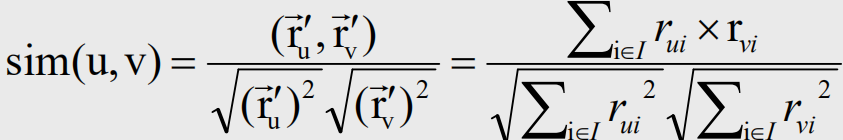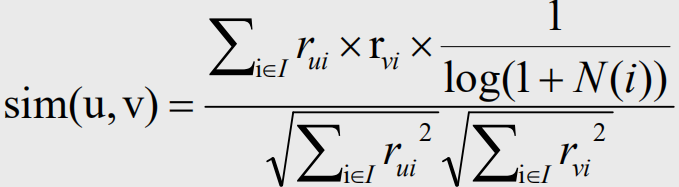### 3.2 ItemCF算法变种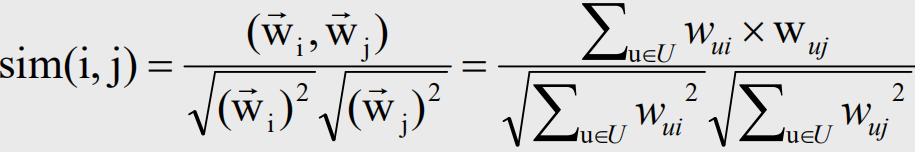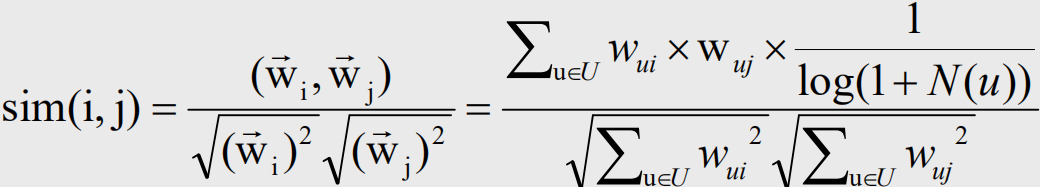03-106207
11-284506

03-01
02-033549
09-29
09-301185
03-232万+
03-093509
07-208万+
07-07

### “相关推荐”对你有帮助么？

•非常没帮助
•没帮助
•一般
•有帮助
•非常有帮助¥2 ¥4 ¥6 ¥10 ¥20余额支付 (余额：-- )扫码支付获取中扫码支付点击重新获取扫码支付1.余额是钱包充值的虚拟货币，按照1:1的比例进行支付金额的抵扣。
2.余额无法直接购买下载，可以购买VIP、C币套餐、付费专栏及课程。余额充值